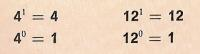The power (2) to printName: ______________________________________  Subject: _______________________ Date: _______

Write on the right side what is missing.

1. Powers of 10

In the upper picture, we see that the powers with the base 10 have a common feature: in all cases the result is the unity (1) followed by a number of zeros determined by the magnitude of the exponent.

Exercises:

 103 = 105 = 102 = 104 = 107 = 106 =2. - Powers of 1 and 0

The power 41 = 4; and 121 = 12. In general, a number raised to the exponent 1 is equal to the same number.

The power 40 = 1 and 120 = 1. A number raised to the exponent 0 is equal to 1.

Exercises:

 1. The power 51 = 2. The power 50 = 3. The power 720 = 4. The power 721 = 5. The power 71 = 6. The power 70 =

3. - Multiplication and division of powers.

The powers of the same base can be multiplied:
34 x 32 = (3 x 3 x 3 x 3) x (3 x 3) = 36.
73 x 72 = (7 x 7 x 7) x (7 x 7) = 75.
To multiply powers with the same base, we write the same base and then we add the exponents.

To divide powers with the same base, we write the same base and then we subtract the exponents.
55 : 52 = (5 x 5 x 5 x 5 x 5) : (5 x 5) = 53.
64 : 62 = (6 x 6 x 6 x 6) : (6 x 6) = 62.

To divide powers with the same base, we write the same base and then we subtract the exponents.

4. - Problems

You can view the topic of how to solve problems

Problems:
 1. You are playing guessing games and Your partner tells you: ōthink a power that is 16 and its base is 2 and then guess the exponentö 2. How much money did a gentleman spend after 7 weeks if he spends 7 euros per day? 3. How many pencils are in 5 boxes that contain 5 bags, if each bag contains 5 pencils? 4. How many erasers are in 12 pencil cases, if each pencil case contains a dozen of erasers? 5. How many trees are in a forest that has 83 lines and 83 trees in each line? 6. Playing guessing games: think a power that is 1000 and its base is 10. What is the exponent?

| Educational applications | Mathematics |
In Spanish  | Interactive

«Arturo Ramo GarcĒa.-Record of intellectual property of Teruel (Spain) No 141, of 29-IX-1999
Plaza Playa de Aro, 3, 1║ DO 44002-TERUEL